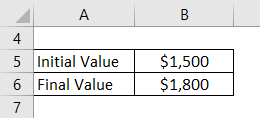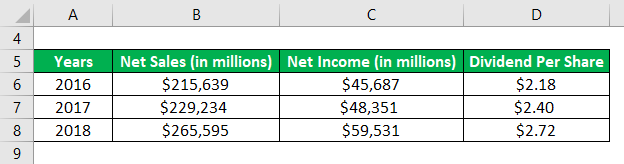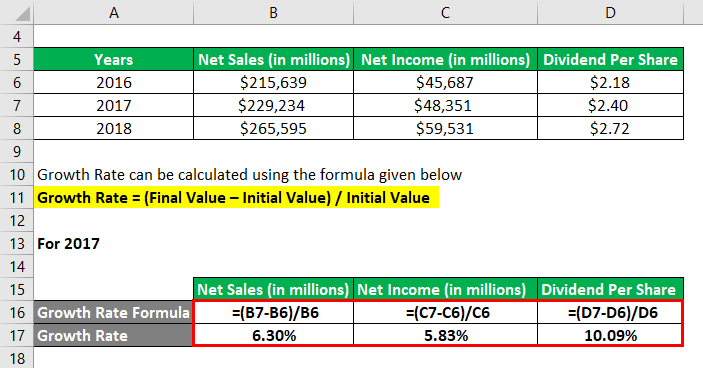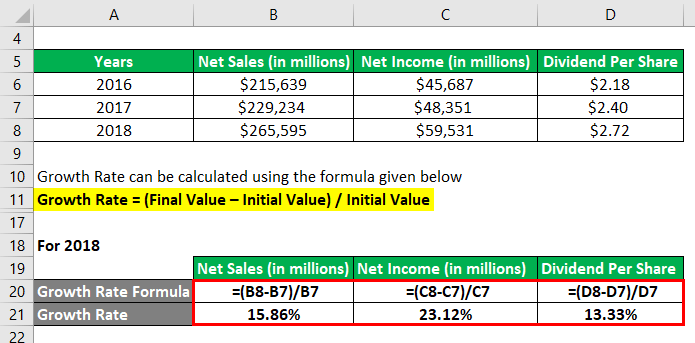# How to Calculate Growth Rate: The Ultimate Guide

Looking how to calculate growth rate for your company? Not sure which method to use? Check out this guide to know everything about calculating growth rate.If you’re running a business, it’s important to keep track of your growth rate. After all, this is one of the key indicators of whether or not your company is doing well. But how do you calculate growth rate? There are actually several different methods for how to calculate growth rate. Which one you use will depend on what data you have available and what makes the most sense for your particular situation. In this guide, we’ll walk you through everything you need to know about how to calculate growth rate so that you can make informed decisions about your business’ future.

## How to Calculate Growth Rate

There are a few different ways to calculate growth rate, but the most common is to take the current period’s growth and divide it by the previous period’s growth. For example, if you’re trying to calculate the quarterly growth rate, you would take the current quarter’s growth and divide it by the previous quarter’s growth.

## What is growth rate?

The growth rate is the rate at which something grows.

The growth rate is a key metric that can help you understand the value of an asset or investment over time. By tracking the growth rate, you can predict how an asset or investment will perform in the future and make informed decisions about where to allocate your resources.

This data can help predict future revenues for a specific company, or a particular stock.

## Key Takeaways Month-Over-Month Growth Metrics

Month-over-month metrics are a great way to measure business growth. However, it’s important to be aware of the difference between linear and compound growth when projecting future performance. In addition to monthly growth rates, it’s important to show the absolute numbers from month to month. This will help prevent overstating the growth rate of the project when it’s still in its infancy.

No matter what kind of business you have, it’s important to keep track of key performance indicators (KPIs). This data can be very helpful in understanding the growth of your business.

As a startup, it’s extremely important to track your growth, even if you aren’t trying to raise capital. Having an understanding of your key metrics such as your MAUs, GM and MRR will help you better manage your team and your company.

Exponential growth models are only as good as the data they’re based on. Month-over-Month growth metrics can give you a solid starting point for your analysis.

## What is the Growth Rate Formula?

The growth rate is the rate at which a quantity changes over a certain time period. This can be applied to any number, including revenue and dividends.

To calculate a growth percentage, you first need to determine the initial and final value of the specific metric you’re measuring. Then, you subtract the beginning value from the end value, and multiply that by 100.

Growth rate = (Final value – Initial value)Initial value

### Examples of Growth Rate Formula (With Excel Template)

Let’s use an example of calculating growth rate to better understand it.

#### Growth Rate Formula – Example #1

If you had invested in a stock exactly one year ago for \$1,500, your investment would currently be worth \$1,800. This represents a growth rate of 20%.

Figure out how much the rate will grow based on the information provided.(Source)

Solution:

The growth rate can be calculated using the equation below.

The growth rate is the difference between the final value (the total number of sales) and the initial (the starting).(Source)

To calculate the growth rate, simply take the difference between the current value and the original value, then divide that number by the original value. In this case, we would take (\$1,800 – \$1,500) to get \$300, and then divide that by \$1,500 to get 0.2. Multiply that number by 100 to get 20%, which is our final answer.

The 20% increase in value means that the company’s stock has grown by 20% over the last year.

#### Growth Rate Formula – Example #2

Apple, Inc., for example, is a great company to look at when examining how a company’s profits, dividends, and sales growth rate increases over time. For the last two years, 2017 and 2018, the company saw growth in all areas.

Does the growth rate of the dividends paid out to shareholders match that of the increase in sales?(Source)

The solution:

The growth rate can be calculated using the equation below.

Growth Rate = (2017 Final Value – 2017 Initial Value) 2017 Initial Value

For 2017(Source)

Total sales

The company’s net sales grew by 6.30% last year.

Net income

The company’s net income grew by 5.83%.

Annual dividend per share

The dividend per share for the company has seen a growth rate of 10.09%. This is calculated by taking the current dividend per share (\$2.40) and subtracting the previous dividend per share (\$2.18). This number is then divided by the previous dividend per share (\$2.18), which gives us the growth rate percentage.

For 2018(Source)

Total sales

The company’s net sales have grown by 15.86%.

Net income

The company’s net income grew by 23.12% last year.

Annual dividend per share

The dividend per share for the company has grown by 13.33%. This is a good sign for the company, as it indicates that they are making more money and can afford to pay out more to shareholders. This growth rate is sustainable in the long term, so investors can expect to see their dividends increase over time.

It is clear to see that the growth rates for Net Sales, Net income, and Dividend Share are all heading in a positive direction. This indicates the company is on a steady and consistent growth path.

What this means is that the company’s dividend growth rate was higher than its net sales growth in 2017, while its revenue growth was slightly higher than its dividend growth rate in 2018.

### Explanation

The formula for calculating your rate of growth is:

First, you’ll need to set a baseline for what you’re measuring. For example, you can use the previous year’s revenue from your financial statements.

Finally, to determine the overall value of this KPI, we will look at how much sales brought in during the previous year.

Step 3: To calculate the change in value, simply deduct the initial value from the final value.

The change in value equals the final value of the investment minus the initial cost.

The growth rate of the metrics can be calculated by dividing the change in the value (Step 3) by the starting value of the metric (Step 1) and then multiplying by 100.

The growth rate is the difference between the final value (the total number of sales) and the initial (the starting).

### The relevance and use of the growth rate formula

The growth rate formula for companies is a simple way for investors and shareholders to gauge how successful a business has been during a certain time period. This calculation is usually made by comparing sales growth, earnings growth, and profit growth in a specific period of time. By understanding this, investors can better assess the health of the company, and predict future successes.

An organization’s ability to grow its sales, earnings, and profits typically is assessed by measuring its growth rate.

Investors can look at either their portfolio’s growth in terms of the book value of their holdings or their returns in terms of the P/E ratio.

Analysts often use two growth measures, Q-O-Q and Y-O-Y, when assessing a company’s performance.

## Why is growth rate important?

The growth rate is the rate at which something is growing. It’s usually represented as a percentage and can tell you a lot about an investment, asset, business, or a portfolio.

The percent change in the market value of a company’s stock from one year to another Growth rate is important because it can help you predict future growth. For example, if the population has been growing by 2% per year, you can predict that it will continue to grow at that rate. Growth rate can also be used to compare different investments or businesses.

Growth rate is important because it helps to indicate how well a business is doing and its expected future growth. The percent change in the overall population from one year to another also helps to show if a business is growing or shrinking.

## What is the formula for rate of growth?

There is no definitive answer to this question as it depends on the specific situation. Generally speaking, the formula for rate of growth is determined by dividing the change in quantity by the change in time. However, there are many factors that can affect this calculation result, such as the starting point, end point, and interval of time considered. As such, it is best to consult with a specialist in order to determine an accurate formula for rate of growth.

### How do you calculate growth rate per year?

There are a few different ways to calculate growth rate per year. One way is to take the current year’s value and divide it by the previous year’s value. This will give you the percentage of growth from one year to the next. Another way to calculate growth rate is to take the difference between two years and divide it by the number of years between them. This will give you the average annual growth rate over a period of time.

## Conclusion

There are several different methods for calculating growth rate, and which one you use will depend on what

growth data you have available and what makes the most sense for your particular situation. In this guide, we’ve walked you through everything you need to know about how to calculate growth rate so that you can make informed monetary policy decisions about your business’s future.